# Important questions Class 10 Maths Chapter 6-Triangles

Important questions for Class 10 Maths Chapter 6 Triangles are provided here to help the students in their exam preparation based on the new pattern of CBSE for 2019-2020 academic session. Students who are preparing for the board exams of 2020 are advised to practice these important questions of Triangles to score full marks for the questions from this chapter. They can also access important questions for 10th maths all chapters at BYJU’S.

The chapter Triangles contains many topics related to a triangle such as criteria for similarity, congruency, areas of similar triangles and Pythagoras theorem, etc., Students can also get the solutions for all the questions of Class 10 Maths of NCERT textbook.

## Important Questions & Answers For Class 10 Maths Chapter 6 Triangles

Q. 1: In the given figure, PS/SQ = PT/TR and ∠ PST = ∠ PRQ. Prove that PQR is an isosceles triangle.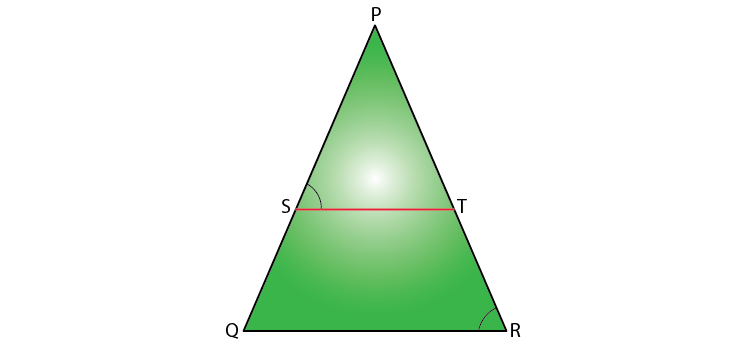Solution:

Given,

PS/SQ = PT/TR

We know that if a line divides any two sides of a triangle in the same ratio, then the line is parallel to the third side.

Therefore, ST // QR

And ∠ PST = ∠ PQR (Corresponding angles) ……..(i)

∠ PST = ∠ PRQ (given( ………(ii)

From (i) and (ii),

∠ PRQ = ∠ PQR

Therefore, PQ = PR (sides opposite the equal angles)

Hence, PQR is an isosceles triangle.

Q. 2: In the figure, DE // AC and DF // AE. Prove that BF/FE = BE/EC.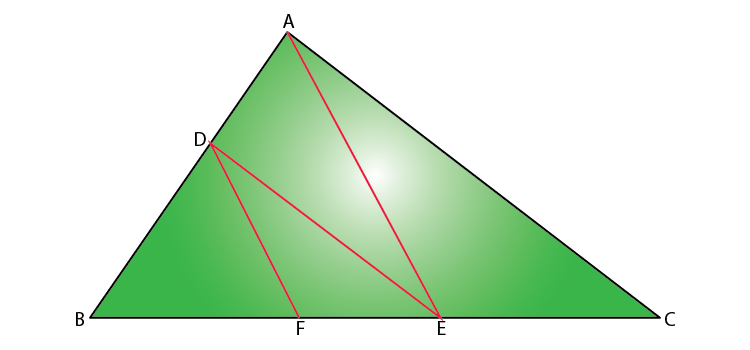Solution:

Given that,

In triangle ABC, DE // AC.

By Basic Proportionality Theorem,

BD/DA = BE/EC……….(i)

Also, given that DF // AE.

Again by Basic Proportionality Theorem,

BD/DA = BF/FE……….(ii)

From (i) and (ii),

BE/EC = BF/FE

Hence proved.

Q. 3: In the given figure, altitudes AD and CE of ∆ ABC intersect each other at the point P. Show that:

(i) ∆AEP ~ ∆ CDP

(ii) ∆ABD ~ ∆ CBE

(iv) ∆ PDC ~ ∆ BEC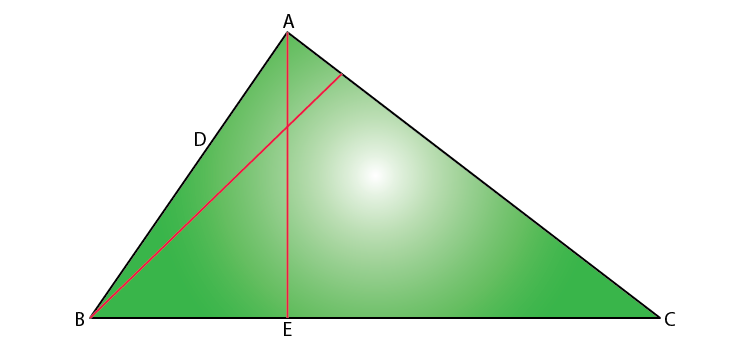Solution:

Given that AD and CE are the altitudes of triangle ABC and these altitudes intersect each other at P.

(i) In ΔAEP and ΔCDP,

∠AEP = ∠CDP (90° each)

∠APE = ∠CPD (Vertically opposite angles)

Hence, by AA similarity criterion,

ΔAEP ~ ΔCDP

(ii) In ΔABD and ΔCBE,

∠ADB = ∠CEB ( 90° each)

∠ABD = ∠CBE (Common Angles)

Hence, by AA similarity criterion,

ΔABD ~ ΔCBE

∠PAE = ∠DAB (Common Angles)

Hence, by AA similarity criterion,

(iv) In ΔPDC and ΔBEC,

∠PDC = ∠BEC (90° each)

∠PCD = ∠BCE (Common angles)

Hence, by AA similarity criterion,

ΔPDC ~ ΔBEC

Q. 4: A vertical pole of length 6 m casts a shadow 4 m long on the ground and at the same time a tower casts a shadow 28 m long. Find the height of the tower

Solution:

Given,

Length of the vertical pole = 6 m

Shadow of the pole = 4 m

Let the height of the tower be h m.

Length of the shadow of the tower = 28 m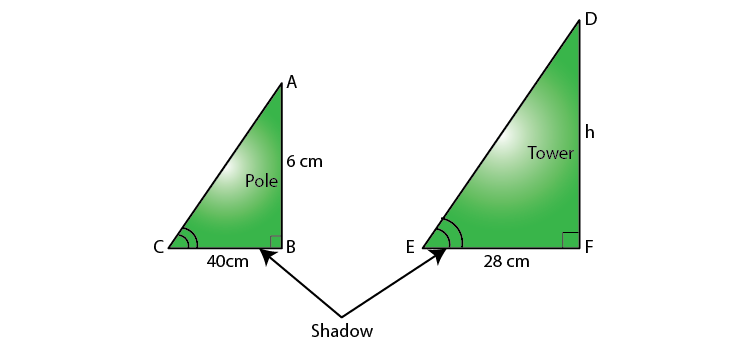In ΔABC and ΔDFE,

∠C = ∠E (angle of elevation)

∠B = ∠F = 90°

By AA similarity criterion,

ΔABC ~ ΔDFE

We know that the corresponding sides of two similar triangles are proportional.

AB/DF = BC/EF

6/h = 4/28

h = (6 ×28)/4

h = 6 × 7

h = 72

Hence, the height of the tower = 42 m.

Q. 5: If ΔABC ~ ΔQRP, ar (ΔABC) / ar (ΔPQR) =9/4 , AB = 18 cm and BC = 15 cm, then PR is equal to

(A) 10 cm (B) 12 cm (C) 20/3 cm (D) 8 cm

Solution:

Given that ΔABC ~ ΔQRP.

ar (ΔABC) / ar (ΔPQR) =9/4

AB = 18 cm and BC = 15 cm

We know that the ratio of the areas of two similar triangles is equal to the square of the ratio of their corresponding sides.

ar (ΔABC) / ar (ΔPQR) = BC2/RP2

9/4 = (15)2/RP2

RP2 = (4/9) ×225

PR2 = 100

Therefore, PR = 10 cm

Q. 6: If the areas of two similar triangles are equal, prove that they are congruent.

Solution:

Let ΔABC and ΔPQR be the two similar triangles with equal area.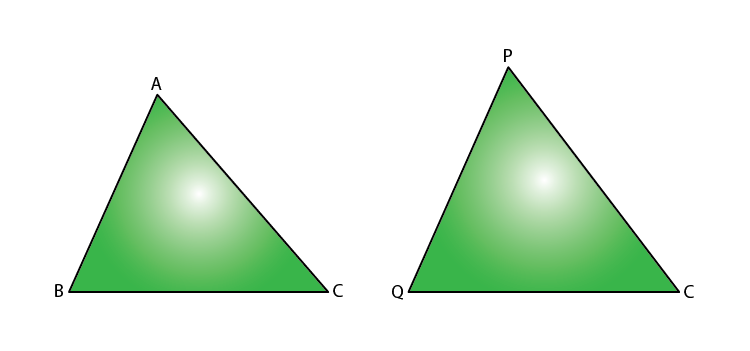To prove ΔABC ≅ ΔPQR.

Proof:

ΔABC ~ ΔPQR

∴ Area of (ΔABC)/Area of (ΔPQR) = BC2/QR2

⇒ BC2/QR2 =1 [Since, ar (ΔABC) = ar (ΔPQR)]

⇒ BC2/QR2

⇒ BC = QR

Similarly, we can prove that

AB = PQ and AC = PR

Therefore, ΔABC ≅ ΔPQR [SSS criterion of congruence]

Q. 7: O is any point inside a rectangle ABCD as shown in the figure. Prove that OB2 + OD2 = OA2 + OC2.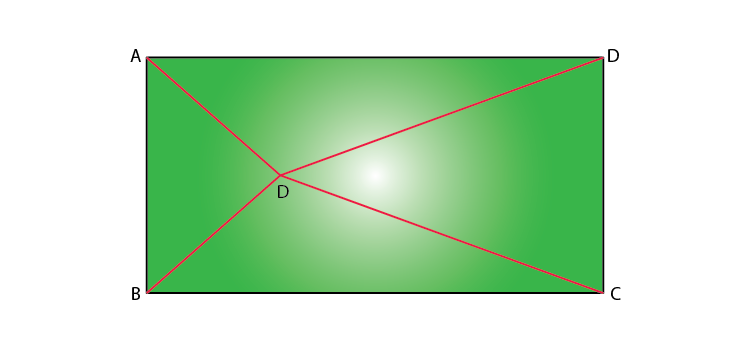Solution:

Through O, draw PQ || BC so that P lies on AB and Q lies on DC.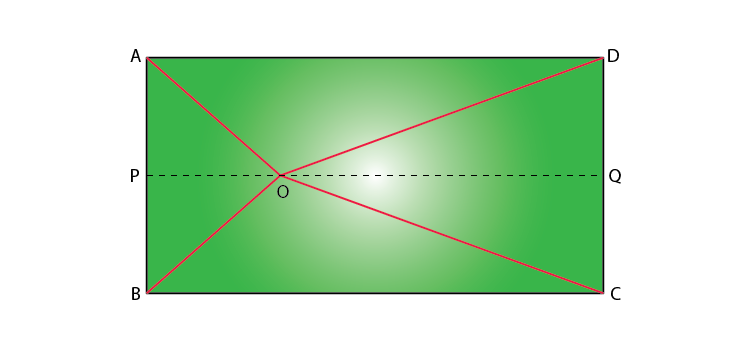PQ || BC

Therefore, PQ ⊥ AB and PQ ⊥ DC (∠ B = 90° and ∠ C = 90°)

So, ∠ BPQ = 90° and ∠ CQP = 90°

Hence, BPQC and APQD are both rectangles.

By Pythagoras theorem,

In ∆ OPB,

OB2 = BP2 + OP2…..(1)

Similarly,

In ∆ OQD,

OD2 = OQ2 + DQ2…..(2)

In ∆ OQC,

OC2 = OQ2 + CQ2…..(3)

In ∆ OAP,

OA2 = AP2 + OP2…..(4)

OB2+ OD2 = BP2 + OP2 + OQ2 + DQ2

= CQ2 + OP2 + OQ2 + AP2

(since BP = CQ and DQ = AP)

= CQ2 + OQ2 + OP2 + AP2

= OC2 + OA2 [From (3) and (4)]

Hence proved that OB2 + OD2 = OA2 + OC2.

Q. 8: Sides of triangles are given below. Determine which of them are right triangles.

In case of a right triangle, write the length of its hypotenuse.

(i) 7 cm, 24 cm, 25 cm

(ii) 3 cm, 8 cm, 6 cm

Solution:

(i) Given, sides of the triangle are 7 cm, 24 cm, and 25 cm.

Squaring the lengths of the sides of the, we will get 49, 576, and 625.

49 + 576 = 625

(7)2 + (24)2 = (25)2

Therefore, the above equation satisfies Pythagoras theorem. Hence, it is a right-angled triangle.

Length of Hypotenuse = 25 cm

(ii) Given, sides of the triangle are 3 cm, 8 cm, and 6 cm.

Squaring the lengths of these sides, we will get 9, 64, and 36.

Clearly, 9 + 36 ≠ 64

Or, 32 + 62 ≠ 82

Therefore, the sum of the squares of the lengths of two sides is not equal to the square of the length of the hypotenuse.

Hence, the given triangle does not satisfy Pythagoras theorem.

### Practice Questions For Class 10 Maths Chapter 6 Triangles

1. Corresponding sides of two similar triangles are in the ratio of 2 : 3. If the area of the smaller triangle is 48 cm2, find the area of the larger triangle.
2. A foot of a 10 m long ladder leaning against a vertical wall is 6 m away from the base of the wall. Find the height of the point on the wall where the top of the ladder reaches.
3. An aeroplane leaves an airport and flies due north at a speed of 1000 km per hour. At the same time, another aeroplane leaves the same airport and flies due west at a speed of 1200 km per hour. How far apart will be the two planes after 1 1/2 hours?
4. Diagonals AC and BD of a trapezium ABCD with AB || DC intersect each other at the point O. Using a similarity criterion for two triangles, show that OA/OC= OB/OD.
5. Prove that if in a triangle square on one side is equal to the sum of the squares on the other two sides, then the angle opposite the first side is a right angle.

1. dimple